envelope

Signal envelope

Description

example

[yupper,ylower] = envelope(x) returns the upper and lower envelopes of the input sequence, x, as the magnitude of its analytic signal. The analytic signal of x is found using the discrete Fourier transform as implemented in hilbert. The function initially removes the mean of x and adds it back after computing the envelopes. If x is a matrix, then envelope operates independently over each column of x.

example

[yupper,ylower] = envelope(x,fl,'analytic') returns the envelopes of x determined using the magnitude of its analytic signal. The analytic signal is computed by filtering x with a Hilbert FIR filter of length fl. This syntax is used if you specify only two arguments.

example

[yupper,ylower] = envelope(x,wl,'rms') returns the upper and lower root-mean-square envelopes of x. The envelopes are determined using a sliding window of length wl samples.

example

[yupper,ylower] = envelope(x,np,'peak') returns the upper and lower peak envelopes of x. The envelopes are determined using spline interpolation over local maxima separated by at least np samples.

example

envelope(___) with no output arguments plots the signal and its upper and lower envelopes. This syntax accepts any of the input arguments from previous syntaxes.

Examples

collapse all

Generate a quadratic chirp modulated by a Gaussian. Specify a sample rate of 2 kHz and a signal duration of 2 seconds.

t = 0:1/2000:2-1/2000;
plot(t,q)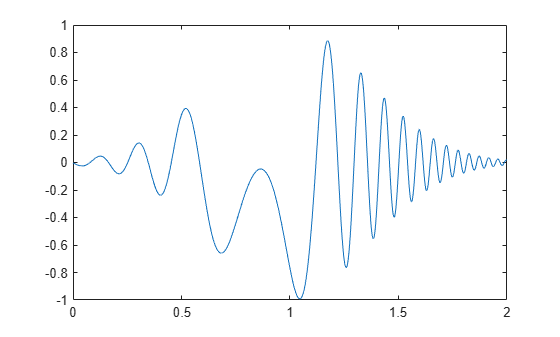Compute the upper and lower envelopes of the chirp using the analytic signal.

[up,lo] = envelope(q);
hold on
plot(t,up,t,lo,'linewidth',1.5)
legend('q','up','lo')
hold offThe signal is asymmetric due to the nonzero mean.

Use envelope without output arguments to plot the signal and envelopes as a function of sample number.

envelope(q)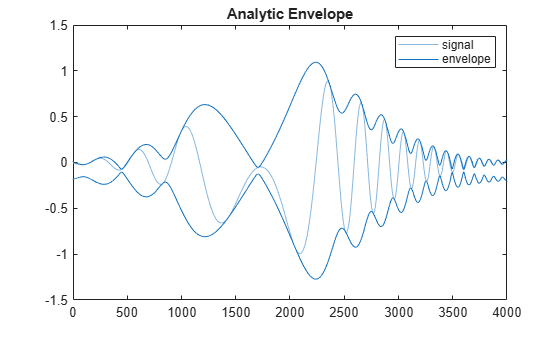Create a two-channel signal sampled at 1 kHz for 3 seconds:

• One channel is an exponentially decaying sinusoid. Specify a frequency of 7 Hz and a time constant of 2 seconds.

• The other channel is a time-displaced Gaussian-modulated chirp with a DC value of 2. Specify an initial chirp frequency of 30 Hz that decays to 5 Hz after 2 seconds.

Plot the signal.

t = 0:1/1000:3;
q1 = sin(2*pi*7*t).*exp(-t/2);
q2 = chirp(t,30,2,5).*exp(-(2*t-3).^2)+2;
q = [q1;q2]';

plot(t,q)Compute the upper and lower envelopes of the signal. Use a Hilbert filter with a length of 100. Plot the channels and the envelopes. Use solid lines for the upper envelopes and dashed lines for the lower envelopes.

[up,lo] = envelope(q,100,'analytic');
hold on
plot(t,up,'-',t,lo,'--')
hold offCall envelope without output arguments to produce a plot of the signal and its envelopes as a function of sample number. Increase the filter length to 300 to obtain a smoother shape. The 'analytic' flag is the default when you specify two input arguments.

envelope(q,300)Compute and plot the moving RMS envelopes of a recording of a train whistle. Use a window with a length of 150 samples.

envelope(y,150,'rms')Plot the upper and lower peak envelopes of a speech signal smoothed over 30-sample intervals.

envelope(mtlb,30,'peak')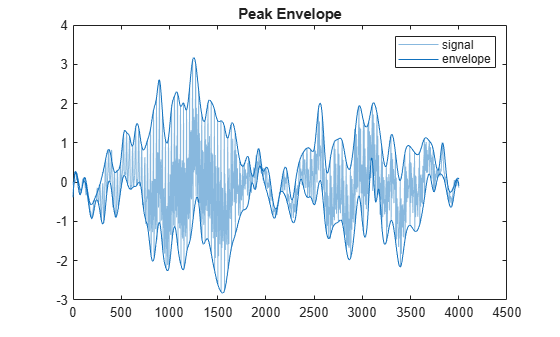Create and plot a signal that resembles the initial detection of a light pulse propagating through a dispersive medium.

t = 0.5:-1/100:-2.49;
z = airy(t*10).*exp(-t.^2);

plot(z)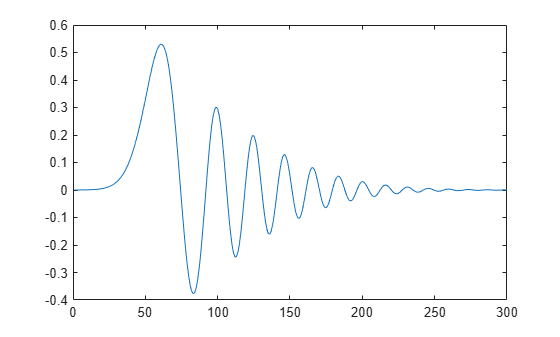Determine the envelopes of the sequence using the magnitude of its analytic signal. Plot the envelopes.

envelope(z)Compute the analytic envelope of the signal using a 50-tap Hilbert filter.

envelope(z,50,'analytic')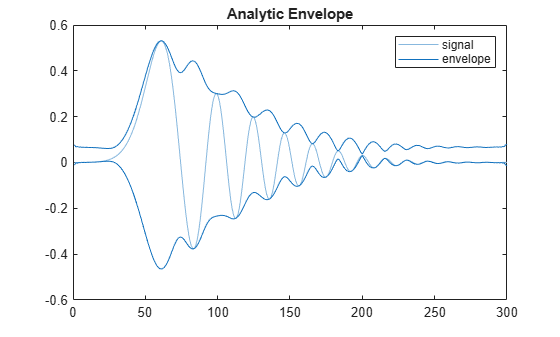Compute the RMS envelope of the signal using a 40-sample moving window. Plot the result.

envelope(z,40,'rms')Determine the peak envelopes. Use spline interpolation with not-a-knot conditions over local maxima separated by at least 10 samples.

envelope(z,10,'peak')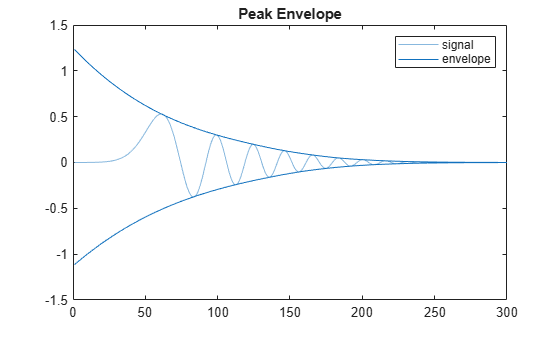Input Arguments

collapse all

Input sequence, specified as a vector or matrix. If x is a vector, it is treated as a single channel. If x is a matrix, then envelope computes the envelope estimates independently for each column. All elements of x must be finite.

Example: cos(pi/4*(0:159))+randn(1,160) is a single-channel row-vector signal.

Example: cos(pi./[4;2]*(0:159))'+randn(160,2) is a two-channel signal.

Data Types: single | double

Hilbert filter length, specified as a positive integer scalar. The filter is created by windowing an ideal brick-wall filter with a Kaiser window of length fl and shape parameter β = 8.

Data Types: single | double

Window length, specified as a positive integer scalar.

Data Types: single | double

Peak separation, specified as a positive integer scalar.

Data Types: single | double

Output Arguments

collapse all

Upper and lower signal envelopes, returned as vectors or matrices.